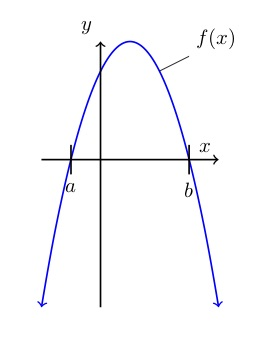# What’s up (more), the parabola or the line?

## QuestionThe graph to the left shows the function f(x), a parabola – that is, a quadratic function. Not shown on the graph is function  in which c is a negative constant.

One statement below is true. Which one?

## Solution

What’s going on? The line will cross the parabola in a couple of places and those intersections will determine which function is higher in various places. But all you know about function is that  and that c is negative.

is the equation of a straight line. Think of a line’s basic equation:  where b is the line’s y-intercept – that is, y’s value where . In this case, because  nothing, the y-intercept is 0. That means the line goes through the origin.

Also, you’re told that c is negative. c is in the role of m in the equation that is, it’s the slope. The negative slope means this line goes down as it goes to the right.

How steep is the line? You are given no information about that. Take a guess.

The brown line in the graph to the right shows what function g may look like. It may be steeper or less steep than shown,but it will cross the x-axis at the origin, and it will go down as it goes to the right, as shown.

Let’s go through all the possible answers to find the one true statement.

1. . At , as you can see from the graph, the parabola is above the x-axis, which means f(0) is a positive value. The line goes right through the origin – so . Thus which means it cannot be true that .
2. . At , function f crosses the x-axis. So . At , function g crosses the x-axis. So . That means f(a) is equal to g(0), so it’s not possible that f(a) is less than g(0).
3. . What’s happening at ? Function f crosses the x-axis there, so ; and function g has a positive value. That means g is more than f, so it must be that . Looks like that’s the answer, but let’s still check the others.
4. . We have established that . And since g(x) goes through the origin and slopes down, g must be less than zero at any x-value that is greater than 0 – like, for example, b. That means g is less than f, so it is not possible that f is less than g.
5. . As you can see from the graph, . And, as we said in response to answer #4, g(b) is less than 0. So f(b) must be more than g(b) – and it’s not possible that .

So answer 3, and only answer 3, works.

I found this question intimidating, because it seems so difficult to know anything about function g. But once you look closely, it’s not so bad. The key is to notice that g takes on positive values to the left of and negative values to the right of .